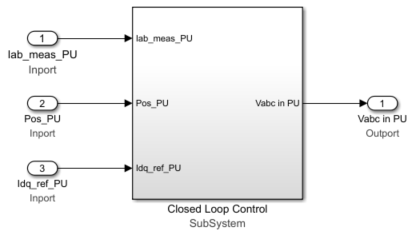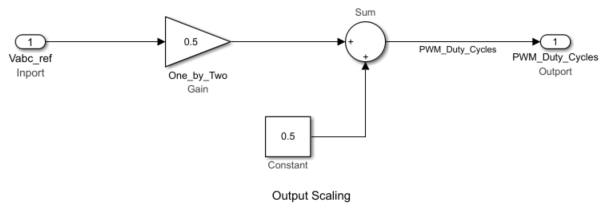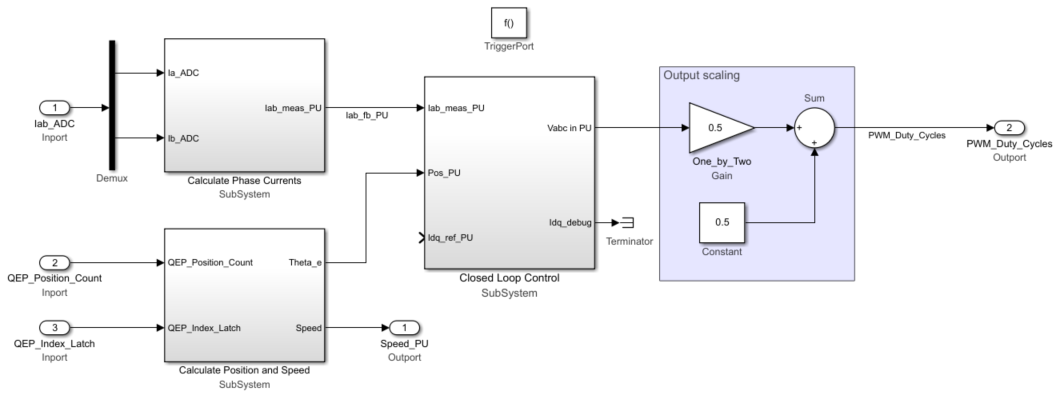## Design Current Controller Subsystem

Use these steps to design the current controller subsystem:

1. From the Motor Control Blockset™ library in the Simulink® Library Browser, use the Discrete PI Controller with anti-windup & reset block (in the Controls/Controllers library) to design the d-axis and q-axis current control.The MATLAB function `mcb.internal.SetControllerParameters` (in the model initialization script) calculates the PI control gains for the d-axis and q-axis current controller and speed controller. For details regarding the control parameter gain estimation, see Estimate Control Gains from Motor Parameters. See the model initialization script file `mcb_pmsm_foc_qep_f28379d_data.m` (used in the example Field-Oriented Control of PMSM Using Quadrature Encoder) for the sampling time (Ts) of `50`μs.

In the subsystem diagram, the Enable variable is a Data Store Memory used to reset the controller. Adding Enable variable is optional.

The subsystem also uses three constant blocks with these values:

• PI_params.Kp_i

• PI_params.Ki_i*Ts

• 0

Create a similar subsystem for the q-axis PI controller. Integrate the subsystems for d-axis and q-axis PI controllers into a single subsystem (Current_Controllers) that controls the d-axis and q-axis currents.

2. Add the Clarke Transform, Park Transform, Inverse Park Transform, and Space Vector Generator blocks from the ```Motor Control Blockset/Controls/Math Transforms``` library to the Current_controllers subsystem (that you created in step 1) as shown in this figure.3. Integrate the components that you created in step 2 into a single subsystem (Closed Loop Control that implements closed loop field-oriented control) as shown in this figure.4. Create an Output scaling subsystem to scale the Pulse Width Modulation (PWM) outputs. This subsystem outputs the normalized PWM duty cycles (`0`-`1`) for the plant model.5. Create a new subsystem by integrating the current scaling, QEP position decoding, Closed Loop Control, and Output scaling subsystems. Add the trigger block from the `Simulink/Ports & Subsystems` library to this subsystem and set the Trigger type parameter to `function-call`.6. Add a Function-Call Generator block from the `Simulink/Ports & Subsystems` library to the subsystem created in step 5. Set the Sample time parameter of the block to equal the control-loop sample time, Ts (that has a default value of `50e-6` s).7. Integrate the plant model and the controller subsystem that you created in step 6. For detailed steps on how to create a plant model for a motor control system, see Creating Plant Model Using Motor Control Blockset.Get ebook